Get instant live expert help with Excel or Google Sheets“My Excelchat expert helped me in less than 20 minutes, saving me what would have been 5 hours of work!”

#### Post your problem and you’ll get Expert help in seconds.

Your message must be at least 40 characters
Our professional Expert are available now. Your privacy is guaranteed.

# How to Find the SUM of a Range with the INDEX Function

We can find the sum of a range with the INDEX function. The steps below will walk through the process.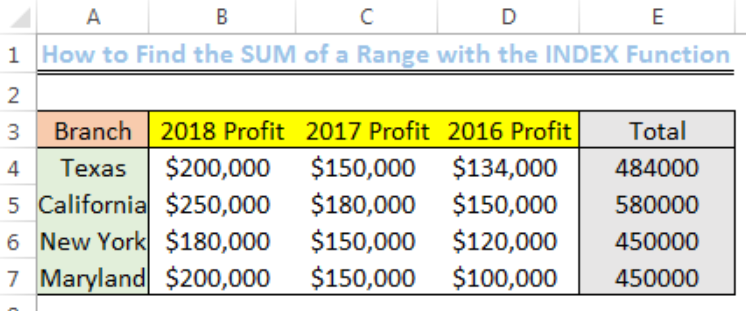Figure 1: Result of the SUM of a Range (THE ROWS) with the INDEX Function

## Setting up the Data

We will set up the data following the scheme in figure 2.

• Cell A4 to Cell A7 will contain the different Branches
• Cell B3, Cell C3, and Cell D3 will contain the profits from 2018 to 2016
• Cell E3 will be titled as TOTAL
• Cell B4 to Cell D7 will contain the profits for each year for the branches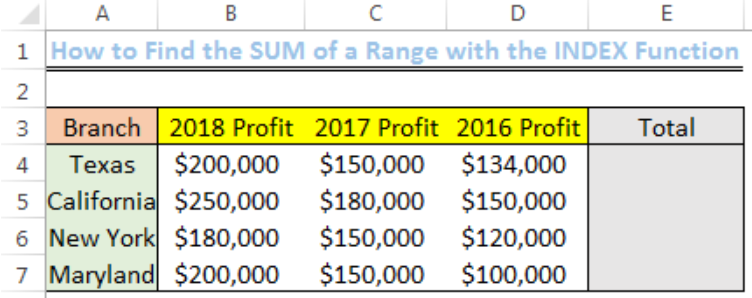Figure 2: How to Find the SUM of a Range with the INDEX Function

## General Formula

`=SUM(INDEX(array,column_number,row_number,))`

Array refers to the range in the spreadsheet that we intend to sum.

## SUM of a Range (ROW) with the INDEX Function

Let us sum the profit for the three years of Texas branch

• We will click on Cell E4 and input the formula below

`=SUM(INDEX(B4:D7,1,0))`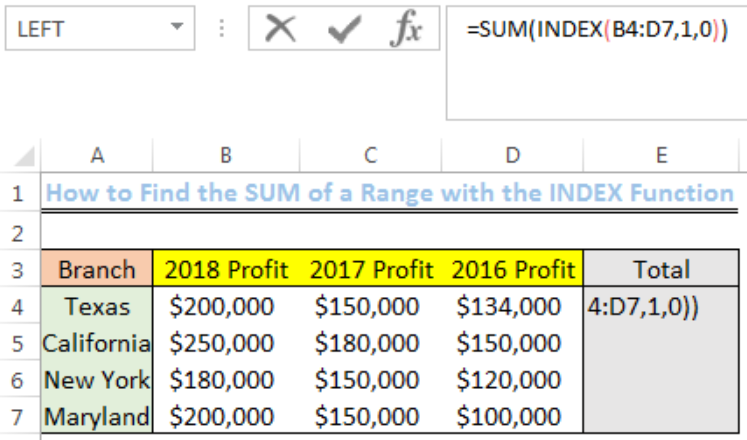Figure 3: SUM of a Range with the INDEX Function

• We will press Enter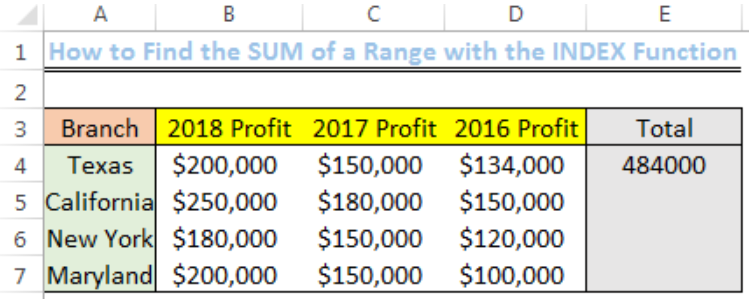Figure 4: SUM of a Range with the INDEX Function

• We will use the drop-down feature to get the sum of the other branches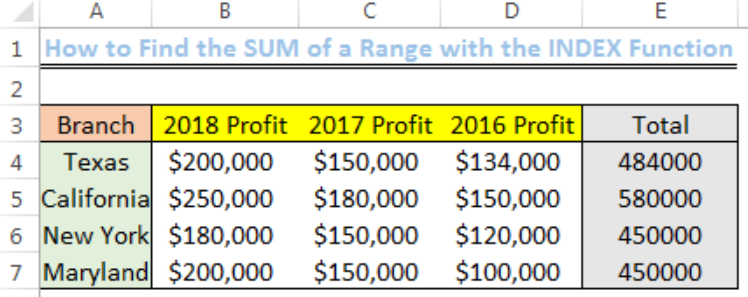Figure 5: Result of the SUM of a Range (THE ROWS) with the INDEX Function

## Explanation

Formula: `=SUM(INDEX(B4:D7,1,0))`

In this formula, the INDEX function returns the row number contained in the range. This happens such that the formula changes in this pattern when the drop-down is used:

`=SUM(INDEX(B4:D7,1,0))`

`=SUM(INDEX(B5:D8,1,0))`

`=SUM(INDEX(B6:D9,1,0))`

`=SUM(INDEX(B7:D10,1,0))`

After the INDEX function returns the values in the row of the range, the SUM function adds the values and the result is displayed.

## Note

If we want to sum columns rather than rows, we will alter the position of the zero and one in the formula.

The formula becomes:

`=SUM(INDEX(B4:D7,0,1))`

## Instant Connection to an Expert through our Excelchat Service

Most of the time, the problem you will need to solve will be more complex than a simple application of a formula or function. If you want to save hours of research and frustration, try our live Excelchat service! Our Excel Experts are available 24/7 to answer any Excel question you may have. We guarantee a connection within 30 seconds and a customized solution within 20 minutes.

### Did this post not answer your question? Get a solution from connecting with the expert.Another blog reader asked this question today on Excelchat:
Solution examplescould someone breakdown this formula for me :D =ArrayFormula(IF(D\$5>=ROWS(B\$5:B5),INDEX(Sheet1!C\$6:C\$21,SMALL(IF(Sheet1!D\$6:D\$21=\$E\$5,ROW(Sheet1!C\$6:C\$21)-ROW(Sheet1!C\$6)+1),ROWS(B\$5:B5))),""))
Solved by C. C. in 40 minsI need a formula to calculate the MAX of a range of 167 rows in column D, then find the MAX of the next 167 rows in column D and so on continuously through the entire sheet. Example MAX D2:D169, D170:D337, D338:D505 etc...
Solved by I. Q. in 60 minsI need a formula that will check if 2 or more cells containing names match then add the adjacent cells to matching name together.
Solved by F. D. in 59 minsHow can I automatically change different cells and the information they display if we select different option from a drop down list?
Solved by V. U. in 59 minsI need to create a rows in Sheet 1 where Sheet 2 column c has values in Sheet 2 column D that are greater than .01 and leave out items in Sheet 1 where Sheet 3 column D are = 0 (without using a filter, then copy/paste).
Solved by I. Y. in 21 mins## Subscribe to Excelchat.coAnother blog reader asked this question today on Excelchat: